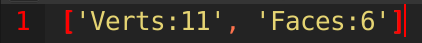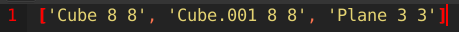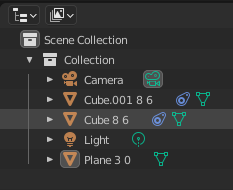# How to copy the statistics info?

Also, how can I display it as a list for each selected object? (so i dont need to run the code 50 times for each object) This would be a big help.

Sure.``````import bpy

VAR_LIST = []

c1 = bpy.context.window_manager.clipboard = str(bpy.context.scene.statistics(bpy.context.view_layer))
c1.encode("utf8")
for c2 in c1.split(" | "):
if "Verts:" in c2 or "Faces" in c2:
VAR_LIST.append(c2)

bpy.context.window_manager.clipboard = str(VAR_LIST)
bpy.ops.text.new()
bpy.ops.text.paste()
``````
1 Like

Yes you can do this, but since it’s object oriented programming (OOP), you’re going to need to access each object individually.

``````import bpy

VAR_LIST = []

c1 = bpy.context.window_manager.clipboard = str(bpy.context.scene.statistics(bpy.context.view_layer))
c1.encode("utf8")

for o in bpy.data.objects:
if o.type == "MESH":
m = o.evaluated_get(bpy.context.evaluated_depsgraph_get()).to_mesh()
c1 = str(str(o.name) + " | Vertices | " + str(len(m.vertices)) + " | Faces | " + str(str(len(m.vertices))))
VAR_LIST.append(c1)

bpy.context.window_manager.clipboard = str(VAR_LIST)
bpy.ops.text.new()
bpy.ops.text.paste()
``````
1 Like

thank you very much. You saved me hours of work.
I have one last question on that.
I want the vertices and faces added to the name to each object. So that “object1” becomes “object1 8512 8512”. Can you add that to the code?

Done. I also added spaces between the object name, vertices count & faces count to help distinguish between each of the 3 type of strings.

# Screenshot# Script

``````import bpy

VAR_LIST = []

c1 = bpy.context.window_manager.clipboard = str(bpy.context.scene.statistics(bpy.context.view_layer))
c1.encode("utf8")

for o in bpy.data.objects:
if o.type == "MESH":
m = o.evaluated_get(bpy.context.evaluated_depsgraph_get()).to_mesh()
c1 = str(str(o.name) + " " + str(len(m.vertices)) + " " + str(str(len(m.vertices))))
VAR_LIST.append(c1)

bpy.context.window_manager.clipboard = str(VAR_LIST)
bpy.ops.text.new()
bpy.ops.text.paste()
``````
1 Like

I mean adding it to the object name
in the outliner

Done… I removed the copy/paste code though. If you want it re-implemented, you’ll need to tell me which one you want re-included. It’s getting a bit difficult to keep track of which one you’re keeping.

# Screenshot# Script

``````import bpy

for o in bpy.data.objects:
if o.type == "MESH":
m = o.evaluated_get(bpy.context.evaluated_depsgraph_get()).to_mesh()
o.name = str(str(o.name) + " " + str(len(m.vertices)) + " " + str(str(len(m.vertices))))
``````
1 Like

thank you. that is the right code, but instead of 2x vertices, i need the vertices and then the faces numbers. So e.g.:
Objekt 500 502

My apologies! For some reason the second object attribute was set to vertices as well, not faces.Should be fixed now. Feel free to tell me if it isn’t.

# Screenshot# Script

``````import bpy

for o in bpy.data.objects:
if o.type == "MESH":
m = o.evaluated_get(bpy.context.evaluated_depsgraph_get()).to_mesh()
o.name = str(str(o.name) + " " + str(len(m.vertices)) + " " + str(len(m.polygons)))
``````
1 Like

Thank you so much!